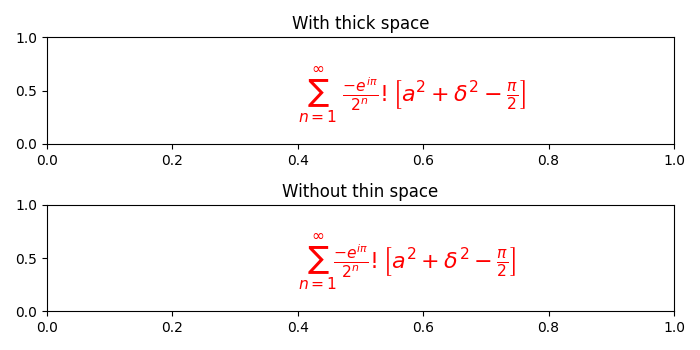# How to remove random unwanted space in LaTeX-style maths in matplotlib plot?

MatplotlibPythonData Visualization

LaTeX ignores the spaces you type and uses spacing the way it's done in mathematics texts. You can use the following four commands in case you want a different spacing style

• \; – thick space
• \: – medium space
• \, – a thin space
• \! – a negative thin space

To remove random unwanted space in LaTeX-style maths in matplotlib plot, we can use "\!" which will reduce the extra spacing.

Let's take an example and understand how it works. We will have two sub-plots and then we will add a complex mathematical equation (using LaTex) in a textbox in both the sub-plots. However, we will use a thick space in one equation and replace it with a thin space in the other equation to show how they appear on the output screen.

## Example

from matplotlib import pyplot as plt

plt.rcParams["figure.figsize"] = [7.00, 3.50]
plt.rcParams["figure.autolayout"] = True

plt.subplot(211)
plt.text(0.4, 0.4, r'$\sum_{n=1}^{\infty}\; \frac{-e^{i\pi}}{2^n}!\left [a^2+\delta ^2- \frac{\pi}{2} \right ]$', fontsize=16, color='r')
plt.title("With thick space")

plt.subplot(212)
plt.text(0.4, 0.4, r'$\sum_{n=1}^{\infty}\! \frac{-e^{i\pi}}{2^n}!\left [a^2+\delta ^2- \frac{\pi}{2} \right ]$', fontsize=16, color='r')
plt.title("With thin space")

plt.show()

## Output

It will produce the following outputNotice the difference in spacing after the "Σ (sigma)" symbol. In the first case, we have used thick space (\;) and in the second case, we have used the thin space (\!) to reduce extra spacing.